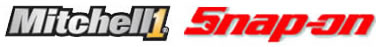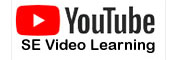Profit WizardThe Profit Wizard provides you with a graphical representation of your profit margin on the order in the form of a pie chart and a summary of the profit detail in the form of the Summary Detail matrix.

To access the Profit Wizard:

• Click [F12] in any open order.

The cost elements of the profitability calculation displayed in the Profit Wizard are described below:

• Labor Cost-  The estimated cost of labor as a percentage of the order total.
• Sublet Cost- The estimated cost of sublet operations as a percentage of the order total.
• Parts Cost- The estimated cost of parts as a percentage of the order total.
• Misc- Miscellaneous Overhead as a fixed percentage of the order total